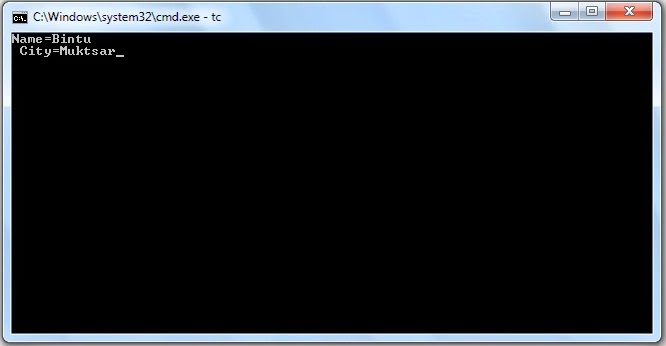## Monday, March 31, 2014

### Simple Structure Program

 Home � C Structures � Simple Structure ProgramNote:- struct is the keyword which we can't use as a variable.

### Pointer Program Using Function (Pass By Reference)

 Home � C Pointers � Pointer Program Using Function (Pass By Reference)

### Pointer Program

 Home � C Pointers � Pointer

### Recursion Function

 Home � C Functions � Recursion Function

### Function with argument and return value

 Home � C Functions � Function with argument and return value

Output is as:

Enter the Two No. for Sum :
10
10
Sum= 20

### Function with no argument and return value

 Home � C Functions � Function with no argument and return value

Output is as:

Enter the Two No. for Sum :
10
10
Sum= 20

### Function with argument and no return value

 Home � C Functions � Function with argument and no return value

Output is as:

Enter the Two No. for Sum :
10
10
Sum= 20

### Function with no argument and no return value.

 Home � C Functions � Function with no argument and no return value

Output is as:

Enter the Two No. for Sum :
10
10
Sum= 20

### Program to print a line of text by using the function

 Home � C Functions � A Simple Function

Output

I am a Good Boy

## Friday, March 28, 2014

Output is as :
Enter the Name
Laura
aruaL

### strlen() - String Handling Functions

Output is as :
Enter the Name
Laura
Length of String=5

### strcpy() - String Handling Functions

Output is as :
Enter the two Names
Bintu
Chaudhary
Bintu Chaudhary

### stcmp() - String Handling Functions

Output is as :
Enter the two Names
Bintu
Chaudhary
Both String are not equal

### stcat() - String Handling Functions

Output is as :
Enter the two Names
Bintu
Chaudhary
BintuChaudhary

Output is as :

Output is as :

Output is as :
I=1
I=2
I=3
I=4
I=5
I=7
I=8
I=9
I=10

Output is as :
I=1
I=2
I=3
I=4
I=5

Output is as :
*
**
***
****
*****

Output is as :
I=1
I=2
I=3
I=4
I=5
I=6
I=7
I=8
I=9
I=10

Output is as :
I=1
I=2
I=3
I=4
I=5
I=6
I=7
I=8
I=9
I=10

Output is as :
I=1
I=2
I=3
I=4
I=5
I=6
I=7
I=8
I=9
I=10

## Thursday, March 27, 2014

### Switch Statement

Output is as :
Enter the Choice from Four Days...
S = Sunday
M = Monday
T = Tuesday
H = Thursday

S
Sunday

### Ladder if or else if statement

The marks obtained by a student in 5 different subjects are input through the keyboard. The student gets a division as per the following rules:

=> Percentage above or equal to 60 - First Division
=> Percentage between 50 and 59 - Second Division
=> Percentage between 40 and 49 - Third Division
=> Percentage less than 40 - Fail

Method: 1

Method: 2

### nested if statement

/*If the ages of Ram, sham and Ajay are input through the keyboard, write a program to determine the youngest of the three*/

Output is as :
Enter the three Ages of Ram,Sham and Ajay
14
17
19
Ram is Youngest

## Tuesday, March 25, 2014

### if-else statement - C Programming

/*Any Number is input through the keyboard. write a program to find out whether It is and Odd Number or Even Number.*/

Output is as :
Enter the Number
4
This is Even Number